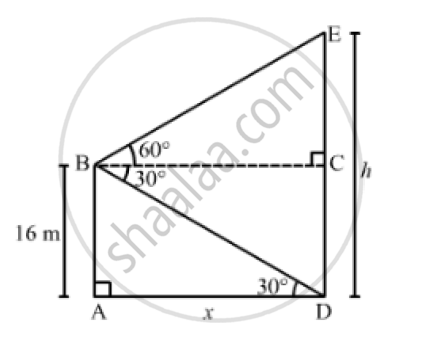# A Man on the Deck of a Ship, 16m Above Water Level, Observe that That Angle of Elevation and Depression Respectively of the Top and Bottom of a Cliff Are 60° and 30° . - Mathematics

A man on the deck of a ship, 16m above water level, observe that that angle of elevation and depression respectively of the top and bottom of a cliff are 60° and 30° . Calculate the distance of the cliff from the ship and height of the cliff.

#### Solution

Let AB be the deck of the ship above the water level and DE be the cliff.

Now,

AB  = 16msuch that CD = 16mand ∠BDA = 30° and ∠EBC = 60°
If AD = xmand DE = hm, then CE = (h-16)m.(AB)/(AD) = tan 30° = 1/ sqrt(3)

⇒16/x = 1/ sqrt(3)

 ⇒x = 16 sqrt(3)=27.68m

In the right ΔEBC,we have:

(EC)/(BC) = tan 60^0 = sqrt(3)

⇒((h-16))/x = sqrt(3)

⇒ h - 16 = xsqrt(3)

⇒ h - 16=16 sqrt(3) xx sqrt(3) = 48                  [ ∵ x = 16 sqrt(3)]

⇒ h = 48+16 = 64 m

∴ Distance of the cliff from the deck of the ship = AD = x = 27.68m

And,
Height of the cliff = DE = h =64m

Concept: Heights and Distances
Is there an error in this question or solution?
Chapter 14: Height and Distance - Exercises

#### APPEARS IN

RS Aggarwal Secondary School Class 10 Maths
Chapter 14 Height and Distance
Exercises | Q 23

Share